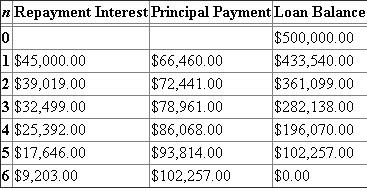# Quiz 15: Capital-Budgeting Decisions

Business

The fifteenth chapter that is in the textbook has to do with various capital budgeting decisions an engineer may have to make for a firm. This includes various ways to finance a project, determination of the cost of capital, and budgeting decisions that may take place. For the problem that we have here on hand, there is a manufacturer of optical storage systems which is the focus of the question at hand. Here they are considering two (2) financing options since they need \$10,000,000.00 for marketing expenses for their new robotic equipment product lines. The one option is to raise the capital through the issuance of capital stock (which has a flotation cost of 6.00% and an initial share price of \$25.00. The other option is debt financing which they could sell a 10-year, 12.00% bond for \$1,000.00. The bond flotation cost there is 1.90%. Given this information, what would the flotation costs be for the equity financing option as well as the number of \$1,000.00 par value bonds that are to be sold for \$10,000,000.00 as well as the applicable interest payment For a problem like this, the answers aren't too hard to calculate as one uses the formulas provided in the text. Let's begin with the first part of the problem which is fairly straightforward to see what is going on. Remember that flotation costs are ones which are expenses for a specific financing option (i.e. marketing of the bond or stock, banker's fees, etc.) that must be added in to the financing option. a) The first question that is on the board asks one to determine the flotation costs for the stock sale and how many shares would need to be sold in order to achieve the \$10,000,000.00 target goal. This has to be done in a couple of steps in order to get the achieved results here. To begin, the first thing one would need to do is to how much the total flotation cost is going to be. The number of shares needing to be sold is denoted by the variable X in the formula below: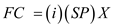….. (1) Where… - FC = the flotation costs, - i = the flotation rate expense applicable here, and - SP = the stock price applicable to the problem. All one would need to do is to add some numbers to the problem so that we can get the appropriate factor here. This is shown below for one to see:FC = \$1.50 X Thus, the flotation cost factor applicable to the problem at hand is approximately \$1.50X. What this means is that this is an immediate deduction off the price of the stock that is of focus here because the net amount is the funding needed for the \$10,000,000.00 goal. The solving continues with a new equation below to get the desired number of shares: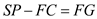….. (2) Where… - FC = the flotation costs, - FG = the financing goal, and - SP = the stock price applicable to the problem. All one would need to do is to add some numbers to the problem so that we can get the appropriate factor here. This is shown below for one to see:X = 425,532 shares of stock Thus, the number of shares that would need to be sold in order to hit the financing target of \$10,000,000.00 would have to be at least 425,532. To get the desired flotation costs here, one would simply need to multiply the number of shares that we just got by the flotation cost factor. When that is done, the net result is \$638,298.00 for the first option. b) The second part of the problem that one would need to solve for here is to determine the flotation costs if one would want to finance the project through debt. As one can see here, the equation that is used here is a little bit different because one would first need to determine the flotation costs that applicable to the problem at hand. Note that the formula shown below is a little different since the flotation cost must be added into the overall financing package to get the desired amount. This and the applicable calculations are shown below for one to see: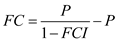….. (3) Where… - FC = the flotation costs, - FCI = the flotation cost interest rate, and - P = the principal amount of the loan needed to be taken out. All one would need to do is to add some numbers to the problem so that we can get the appropriate factor here. This is shown below for one to see:FC = \$193,680.00 Thus, the amount of money that would need to be added to the principal in order to cover costs for the loan is approximately \$193,680.00. Note that the number of bond units this is applicable to is the difference between the total between the principal and flotation costs and then the division by the price of one unit (\$1,000.00). When this is done, there are 10,194 bond units that would need to be sold here. The final thing that one would need to do here is to determine the annual interest payment. All one has to do is to multiply the amount of bond units by the interest rate applicable on the financing (12.00%) and the face value of one bond note (\$1,000.00). When this is done, the annual interest applicable to the cause is \$1,223,280.00.

Present worth or present value is the present prevailing market price of a certain sum ofmoney carrying a required expected rate of return. The G Company makes the computer accessories and the data for the earning per share is given. The company had a long-term debt of \$12,000,000 and paid \$1,200,000 expenses on interest. (a) For the determination of total market value, the corporate value model is used. It is required to determine the logic which the investors use for the assessment of present value. The total market value is the sum of present value of its expected future net cash flows and the value of current assets. In other words, the total market value can be computed as done below:The value of current assets is given to be \$5,000,000. The present value of its expected future net cash flows is computed as done below:(b) It is required to determine the Games' tax rate. It is computed with the formula as shown below:The Games' tax rate is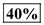. (c) It is needed to do the appropriate MARR when the financing source is known and unknown. Case 1: When the financing is known. In this case, interest rate parity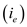is used. The formula for the interest rate parity is shown below: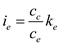By this formula, it can be seen that Common stock is the only source of issuing new stocks. The Debt to equity ratio is computed as shown below:This ratio can be maintained when the new equipment is purchased. Hence, the cost of new equipment will be \$10M, long-term debt of \$14M and equity stock of \$6M. It can be seen that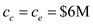. The dividend of the first year is calculated with the formula shown below: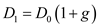where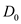is the dividend at the end of the first year from now and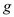is the rate of growth. The growth rate is computed with the formula as shown below:The dividend of the first year is calculated with the formula shown below:The specific cost of the common equity is calculated with the formula as given below: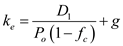Where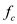is the floating cost and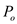is the issuing price.The appropriate MARR is used which is shown below:Hence, the MARR when financing is known is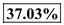. Case 2: When the financing is not known. In this case, weighted average cost of capital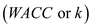is used. The formula for the weighted average cost of capital is shown below: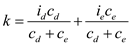Here,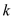is the tax adjusted WACC,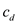is the total debt capital,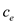is the total equity capital,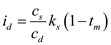is the after-tax average borrowing interest rate which is 10% andis the average equity interest rate per period which is 37.03% as calculated above. This ratio can be maintained when the new equipment is purchased. Hence, the cost of new equipment will be \$10M, long-term debt of \$14M and equity stock of \$6M. It can be seen thatand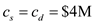. The MARR is computed with the formula as shown below:Hence, the MARR when financing is unknown is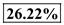. (d) It is required to find the most likely estimate for the Games's stock price. First, it is required to calculate the present worth which can be computed with the appropriate formula as shown below: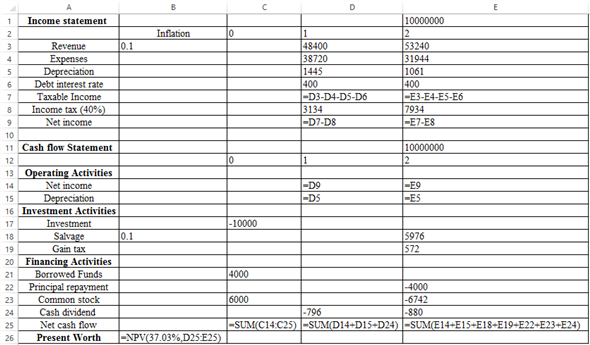The corresponding values are: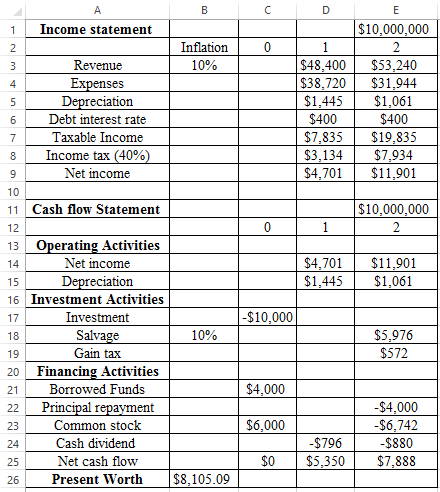The total market value is given to be \$18M. The most likely estimate for Games's stock price is calculated with the formula given below:Hence, the most likely estimate for Games's stock price is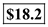. (e) Now, it is assumed that the equipment is disposed of and it is required to finance \$10,000,000. The revenue generated from the new product continue to grow indefinitely. The proper estimate is not being provided for this. So, the pessimistic, most likely and an optimistic estimate cannot be concluded.

The fifteenth chapter that is in the textbook has to do with various capital budgeting decisions an engineer may have to make for a firm. This includes various ways to finance a project, determination of the cost of capital, and budgeting decisions that may take place. For the problem that we have here on hand, we have a company which has an initial investment on a project they are wanting to pursue that costs \$500,000.00 per year at an interest rate of 9.00% annually. Assuming that the interest rate is 9.00%, how would the repayment schedule look like if it was done via equal repayments of the principal, interest, or equalized annual installments While the three (3) questions have some relation to each other, the best thing that one can do here is to independently answer the questions first so that it will make more sense and easier to digest all things considering. Let's look at the questions below and work through the problem in a timely manner. a) The first question that one would need to answer here is to determine the repayment schedule if there was an equal repayment of the principal. Note that this is simply done by dividing up the loan amount (\$500,000.00) into the number of n periods applicable to the situation. Since there are six (6) time periods, the equal amounts of the principal are \$83,333.00. Since there is interest accruing at a rate of 9.00% annually, tis must also be factored in to the problem at hand with an exception. Here, the interest is included with the principal in order to get the desired result. Note that what is shown below is rare because banks would never may any money as of the result: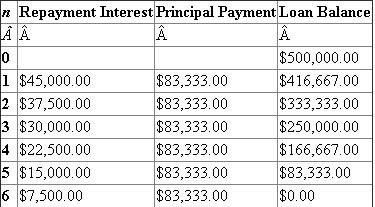b) The second question that one would need to answer here has to do with providing a repayment schedule when the interest payments are identical to each other. Note that the principal is similar in nature as what one has above with the exception that the principal would be paid at the end of the term since the interest payments are identical. Note that in the chart below that the loan balance doesn't move because the interest is cancelled out as it is paid: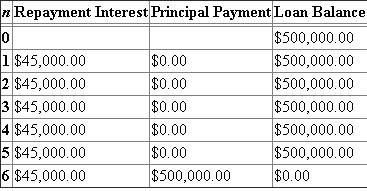Thus, the annual amount repaid in interest is approximately \$45,000.00 per year in this case. c) The final question that one would need to determine here is the amount that would need to be paid if the installments were equalized over the time period mentioned. Note that in order to do this, one would need to apply the following formula and calculations to get the annualized payment below: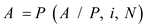….. (1) Where… - P = the present worth of the sum of money in this situation, - A = annual amount placed in the account, - i = interest rate (which is known in this case), and - N = number of terms that the money is for. Since we know the amount of payments but not the annual amount applicable here and the withdrawals that would be taken out of the account once their son becomes of legal age, we would need to apply the values in the formula below to continue on.The next thing that should be done here is to mosey on over to the back of the textbook to get the values for the factor that is shown above. Note that the factor applicable here on the left will be in the sixth column. The factor value and remaining calculations continue below:A = \$111,460.00 (rounded) Thus, the annualized payment that would be applicable to the situation here is approximately \$111,460.00. Note that this amount must be split up between the interest paid on the financing project as well as the principal. Shown below are the applicable numbers for the payoff to occur in six (6) years' time: